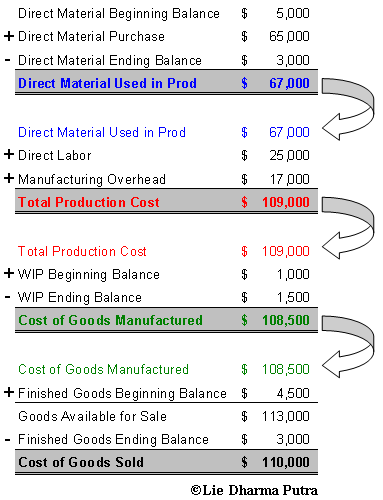Connect with us

# How To Calculate Cost Of Goods Sold (COGS) In Easy WayPublished

on

Do you hard to understand the Cost of Goods Sold flow and its calculation? Not only you, many times we are not given a simple and easy way on how to calculate Cost of Goods Sold right away. Try this way, it may easier as I found it so.

It’s not a rocket science indeed, it is only a matter of moving end calculation of each part (section) to the top of the next part (section). A visual chart may explain better than words, yet, easier to remember. So here is the calculation flow chart:Explanation:

Part-1: Direct Material Usage Calculation

To calculate direct material usage, you need the following information (read: numbers):

1. Direct Material Beginning Balance: On the beginning of a book, you will have beginning balance of “Direct Material Beginning Balance”, it is on the Balance Sheet. If you have not constructed an opening balance sheet, you can find the number on the balance sheet of the previous book. Put this on the top of the section.
2. Direct Material Purchase: This is value of all direct material purchased during the current book period. Note: Don’t forget to include the inbound freight, purchase return and discount in it.
3. Direct Material Ending Balance: This is value of the direct material ending balance after it is used for making the product during the book period.

Have a look the above chart for calculation

Part-2: Product Cost Calculation

To calculate product cost, you need the following information (read: numbers):

1. Direct Material Usage: This number comes from part-1 (see the above chart).
2. Direct Labor Cost: This is the total wages (paycheck) for employees who involved in the process of making the product. They are usually paid by unit product or hours.
3. Manufacturing Overhead: This is total cost incurred to make the product other than the Direct Material and Direct Labor (i.e.: Depreciation of Machineries, Factory Maintenance, Electricity used in the Production, etc.).

See the above chart for calculation
Part-3: Cost of Goods Manufactured Calculation

To calculate cost of goods manufactured, you need the following information (read: numbers):

1. Total Product Cost: This number comes from Part-2.
2. Work In Process (WIP) Beginning Balance: You can get this number from balance sheet of previous book.
3. Wok In process (WIP) Ending Balance: This is value of the work in process ending balance after it is used for making the product during the book period.

Have a look the chart for calculation

Part-4: Cost of Goods Sold Calculation

This is the last part. To calculate the cost of goods sold, you need the following information:

1. Cost of Goods Manufactured: This number comes from Part-3.
2. Finished Goods Beginning Balance: You can get this number from balance sheet of previous book.
3. Finished Goods Ending Balance: This is value of finished goods ending balance after it is sold.

Have a look the chart for calculation.

I hope you find it is useful, and gives an easier understanding on how to calculate cost of goods sold.

Are you looking for easy accounting tutorial? Established since 2007, Accounting-Financial-Tax.com hosts more than 1300 articles (still growing), and has helped millions accounting student, teacher, junior accountants and small business owners, worldwide.